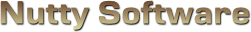High Dynamic Range (HDR)

Sorry, it appears you don't have support for WebGL.

In order to run this demo, you must meet the following requirements.

• You have a WebGL compatible video card with the latest drivers.
• Your video card is not blacklisted. You can check the current blacklist on Khronos.

Some browsers may require additional configuration in order to get WebGL to run. If you are having problems running this demo, visit the following sites.

Use mouse.

#### Luminance

Avg:
Max:

Avg:
Max:
							
/// <summary>
/// The HDR shader doesn't manage lighting. It assumes lighting will be provided as a
/// pre-baked RGBE lightmap. You could optionally support dynamic lighting in your own
/// project as well.
/// </summary>

/// <summary>
/// Material source structure.
/// <summary>
struct MaterialSource
{
vec2 TextureOffset;
vec2 TextureScale;
};

/// <summary>
/// Attributes.
/// <summary>
attribute vec3 Vertex;
attribute vec2 Uv;

/// <summary>
/// Uniform variables.
/// <summary>
uniform mat4 ProjectionMatrix;
uniform mat4 ViewMatrix;
uniform mat4 ModelMatrix;
uniform vec3 ModelScale;

uniform MaterialSource Material;

/// <summary>
/// Varying variables.
/// <summary>
varying vec2 vUv;

/// <summary>
/// <summary>
void main ()
{
// Transform the vertex
gl_Position = ProjectionMatrix * ViewMatrix * ModelMatrix * vec4(Vertex * ModelScale, 1.0);

// Setup the UV coordinates
vUv = Material.TextureOffset + (Uv * Material.TextureScale);
}


							
/// <summary>
/// Vertex shader for rendering a 2D plane on the screen. The plane should be sized
/// from -1.0 to 1.0 in the x and y axis. This shader can be shared amongst multiple
/// </summary>

/// <summary>
/// Attributes.
/// <summary>
attribute vec3 Vertex;
attribute vec2 Uv;

/// <summary>
/// Uniform variables.
/// <summary>
uniform sampler2D Sample0;

/// <summary>
/// Varying variables.
/// <summary>
varying vec2 vUv;
varying vec4 vAvgLuminance;

/// <summary>
/// <summary>
void main ()
{
gl_Position = vec4(Vertex, 1.0);
vUv = Uv;
}


							
/// <summary>
/// The HDR shader doesn't manage lighting. It assumes lighting will be provided as a
/// pre-baked RGBE lightmap. You could optionally support dynamic lighting in your own
/// project as well.
/// </summary>

#ifdef GL_ES
precision highp float;
#endif

/// <summary>
/// Exposure controls the brightness factor of the HDR render.
/// Values greater than 0.0 increase brightness.
/// Values less than 0.0 decrease brightness.
/// <summary>
uniform float Exposure;

/// <summary>
/// Uniform variables.
/// <summary>
uniform sampler2D Sample0;

/// <summary>
/// Varying variables.
/// <summary>
varying vec2 vUv;

/// <summary>
/// ldexp is not part of OpenGL ES 2.0 specification, so it is defined here.
/// <summary>
float ldexp (float x, float exponent)
{
return x * pow(2.0, exponent);
}

/// <summary>
/// frexp is not part of OpenGL ES 2.0 specification, so it is defined here.
/// <summary>
float frexp (float x, out float exponent)
{
exponent = ceil(log2(x));
return(x * exp2(-exponent));
}

/// <summary>
/// Convert a 32 bit RGBE pixel to a 96 bit floating point RGB pixel.
/// <summary>
vec3 RGBEToRGB (vec4 rgbe)
{
if ( rgbe.w > 0.0 )
{
rgbe *= 255.0;
float value = ldexp(1.0, rgbe.w - (128.0 + 8.0));
return rgbe.xyz * value;
}
return vec3(0.0);
}

/// <summary>
/// Convert a 96 bit floating point RGB pixel into a 32 bit RGBE pixel.
/// <summary>
vec4 RGBToRGBE (vec3 rgb)
{
float value = max(max(rgb.x, rgb.y), rgb.z);

if ( value < 0.00001 )
{
return vec4(0.0);
}
else
{
float exponent;
vec4 rgbe = vec4(0.0);
value = frexp(value, exponent) * 256.0 / value;
rgbe.xyz = rgb.xyz * value;
rgbe.w = exponent + 128.0;

return (rgbe / 255.0);
}
}

/// <summary>
/// Bilinearly filter the RGBE texture since this cannot be done in hardware.
/// <summary>
/// <param name="uv">UV coordinates.</param>
/// <returns>Bilinearly filtered RGB pixel.</returns>
vec3 BilinearFilter (vec2 uv)
{
// RGBE textures are 1024x1024
const float ImageSize = 1024.0;
const vec2 TexelSize = vec2(1.0 / ImageSize);

// Readjust the UV to map on the point
vec2 fUv = fract(uv * ImageSize);
uv = floor(uv * ImageSize) / ImageSize;

vec3 tl = RGBEToRGB(texture2D(Sample0, uv));
vec3 tr = RGBEToRGB(texture2D(Sample0, uv + vec2(TexelSize.x, 0.0)));
vec3 bl = RGBEToRGB(texture2D(Sample0, uv + vec2(0.0, TexelSize.y)));
vec3 br = RGBEToRGB(texture2D(Sample0, uv + vec2(TexelSize.x, TexelSize.y)));

vec3 a = mix(tl, tr, fUv.x);
vec3 b = mix(bl, br, fUv.x);
return mix(a, b, fUv.y);
}

/// <summary>
/// <summary>
void main ()
{
// Convert from RGBE to RGB and filter the pixel since the hardware
// can't filter RGBE pixels.
vec3 rgb = BilinearFilter(vUv);

// Apply exposure adjustment to simulate overexposure (such as a bright sun).
// Note that exposure is calculated as 2^exposure. To save on performance, this
// is precalculated in JavaScript.
rgb = rgb * Exposure;

// Reencode to frame buffer as RGBE
gl_FragColor = RGBToRGBE(rgb);
}


							
/// <summary>
/// This shader converts an RGBE image to log luminance. The values are encoded
/// back into RGBE on completion.
/// </summary>

#ifdef GL_ES
precision highp float;
#endif

/// <summary>
/// Uniform variables.
/// <summary>
uniform sampler2D Sample0;

/// <summary>
/// Varying variables.
/// <summary>
varying vec2 vUv;

/// <summary>
/// ldexp is not part of OpenGL ES 2.0 specification, so it is defined here.
/// <summary>
float ldexp (float x, float exponent)
{
return x * pow(2.0, exponent);
}

/// <summary>
/// frexp is not part of OpenGL ES 2.0 specification, so it is defined here.
/// <summary>
float frexp (float x, out float exponent)
{
exponent = ceil(log2(x));
return(x * exp2(-exponent));
}

/// <summary>
/// Convert a 32 bit RGBE pixel to a 96 bit floating point RGB pixel.
/// <summary>
vec3 RGBEToRGB (vec4 rgbe)
{
if ( rgbe.w > 0.0 )
{
rgbe *= 255.0;
float value = ldexp(1.0, rgbe.w - (128.0 + 8.0));
return rgbe.xyz * value;
}
return vec3(0.0);
}

/// <summary>
/// Convert a 96 bit floating point RGB pixel into a 32 bit RGBE pixel.
/// <summary>
vec4 RGBToRGBE (vec3 rgb)
{
float value = max(max(rgb.x, rgb.y), rgb.z);

if ( value < 0.00001 )
{
return vec4(0.0);
}
else
{
float exponent;
vec4 rgbe = vec4(0.0);
value = frexp(value, exponent) * 256.0 / value;
rgbe.xyz = rgb.xyz * value;
rgbe.w = exponent + 128.0;

return (rgbe / 255.0);
}
}

/// <summary>
/// Gets the luminance value for a pixel.
/// <summary>
float GetLuminance (vec3 rgb)
{
// ITU-R BT.709 Primaries
return (0.2126 * rgb.x) + (0.7152 * rgb.y) + (0.0722 * rgb.z);
}

/// <summary>
/// <summary>
void main ()
{
// Add 1.0 to the log luminance because anything less than 1.0
// outputs a negative value, which RGBE doesn't support.
const float delta = 1.0;

// Convert from RGBE to RGB
vec3 rgb = RGBEToRGB(texture2D(Sample0, vUv));

// Get the log luminance value of the pixel
float luminance = GetLuminance(rgb);
float logLuminance = log(delta + luminance);

// Set fragment
gl_FragColor = RGBToRGBE(vec3(logLuminance));
}


							
/// <summary>
/// The role of the mipmap shader is to reduce a texture down to 1x1 in order to find
/// an average or maximum value. To prevent duplicate shader code, this shader uses a
/// define statement to control whether or not this mipmap shader finds the average or
/// the maximum value.
/// </summary>

#ifdef GL_ES
precision highp float;
#endif

/// <summary>
/// This parameter controls whether or not this shader will
/// find the average value or the maximum value between pixels.
/// FIND_TYPE is AVERAGE for finding the average or MAXIMUM to find the maximum.
/// <summary>
#define {FIND_TYPE}

/// <summary>
/// Uniform variables.
/// <summary>
uniform sampler2D Sample0;
uniform vec2 ImageSize;
uniform vec2 TexelSize;
uniform float MipLevelBias;

/// <summary>
/// Varying variables.
/// <summary>
varying vec2 vUv;

/// <summary>
/// ldexp is not part of OpenGL ES 2.0 specification, so it is defined here.
/// <summary>
float ldexp (float x, float exponent)
{
return x * pow(2.0, exponent);
}

/// <summary>
/// frexp is not part of OpenGL ES 2.0 specification, so it is defined here.
/// <summary>
float frexp (float x, out float exponent)
{
exponent = ceil(log2(x));
return(x * exp2(-exponent));
}

/// <summary>
/// Convert a 32 bit RGBE pixel to a 96 bit floating point RGB pixel.
/// <summary>
vec3 RGBEToRGB (vec4 rgbe)
{
if ( rgbe.w > 0.0 )
{
rgbe *= 255.0;
float value = ldexp(1.0, rgbe.w - (128.0 + 8.0));
return rgbe.xyz * value;
}
return vec3(0.0);
}

/// <summary>
/// Convert a 96 bit floating point RGB pixel into a 32 bit RGBE pixel.
/// <summary>
vec4 RGBToRGBE (vec3 rgb)
{
float value = max(max(rgb.x, rgb.y), rgb.z);

if ( value < 0.00001 )
{
return vec4(0.0);
}
else
{
float exponent;
vec4 rgbe = vec4(0.0);
value = frexp(value, exponent) * 256.0 / value;
rgbe.xyz = rgb.xyz * value;
rgbe.w = exponent + 128.0;

return (rgbe / 255.0);
}
}

/// <summary>
/// Gets the luminance value for a pixel.
/// <summary>
float GetLuminance (vec3 rgb)
{
// ITU-R BT.709 primaries
return (0.2126 * rgb.x) + (0.7152 * rgb.y) + (0.0722 * rgb.z);
}

/// <summary>
/// Bilinearly filter the RGBE texture since this cannot be done in hardware.
/// <summary>
/// <param name="uv">UV coordinates.</param>
/// <param name="mipLevelBias">Mipmap bias level.</returns>
/// <returns>Bilinearly filtered RGB pixel.</returns>
vec3 BilinearFilter (vec2 uv, float mipLevelBias)
{
vec2 fUv = fract(uv * ImageSize);
uv = floor(uv * ImageSize) / ImageSize;

vec3 tl = RGBEToRGB(texture2D(Sample0, uv, mipLevelBias));
vec3 tr = RGBEToRGB(texture2D(Sample0, uv + vec2(TexelSize.x, 0.0), mipLevelBias));
vec3 bl = RGBEToRGB(texture2D(Sample0, uv + vec2(0.0, TexelSize.y), mipLevelBias));
vec3 br = RGBEToRGB(texture2D(Sample0, uv + vec2(TexelSize.x, TexelSize.y), mipLevelBias));

vec3 a = mix(tl, tr, fUv.x);
vec3 b = mix(bl, br, fUv.x);
return mix(a, b, fUv.y);
}

/// <summary>
/// Returns the maximum pixel.
/// <summary>
/// <param name="uv">UV coordinates.</param>
/// <param name="mipLevelBias">Mipmap bias level.</returns>
/// <returns>Pixel with the maximum luminosity.</returns>
vec4 MaxFilter (vec2 uv, float mipLevelBias)
{
// Readjust the UV to map on the point
uv = floor(uv * ImageSize) / ImageSize;

// Get rgbe values
vec4 ptl = texture2D(Sample0, uv, mipLevelBias);
vec4 ptr = texture2D(Sample0, uv + vec2(TexelSize.x, 0.0), mipLevelBias);
vec4 pbl = texture2D(Sample0, uv + vec2(0.0, TexelSize.y), mipLevelBias);
vec4 pbr = texture2D(Sample0, uv + vec2(TexelSize.x, TexelSize.y), mipLevelBias);

// Convert to rgb
vec3 tl = RGBEToRGB(ptl);
vec3 tr = RGBEToRGB(ptr);
vec3 bl = RGBEToRGB(pbl);
vec3 br = RGBEToRGB(pbr);

// Get luminance
float ltl = GetLuminance(tl);
float ltr = GetLuminance(tr);
float lbl = GetLuminance(bl);
float lbr = GetLuminance(br);

// Compare luminance and return the brightest one
float maxLuminance = max(max(max(ltl, ltr), lbl), lbr);
if ( ltl == maxLuminance )
return ptl;
else if ( ltr == maxLuminance )
return ptr;
else if ( lbl == maxLuminance )
return pbl;
else
return pbr;
}

/// <summary>
/// <summary>
void main ()
{
#ifdef AVERAGE
vec3 rgb = BilinearFilter(vUv, MipLevelBias);
gl_FragColor = RGBToRGBE(rgb);
#else
gl_FragColor = MaxFilter(vUv, MipLevelBias);
#endif
}


							
/// <summary>
/// The role of the tone map shader is to compress HDR images to LDR before displaying
/// on the monitor. This shader uses the minimal version of Reinhard's tone mapping algorithm.
/// </summary>

#ifdef GL_ES
precision highp float;
#endif

/// <summary>
/// Uniform variables.
/// <summary>
uniform sampler2D Sample0;

/// <summary>
/// Identifies the type of tone mapping algorithm to use.
/// <summary>
uniform int ToneMapAlgorithm;

/// <summary>
/// Stores the average luminance used in the calculation of Reinhard's tonemap.
/// <summary>
uniform float AvgLuminance;

/// <summary>
/// AKA white luminance. Stores the smallest luminance that will be mapped to pure white.
/// Reinhard sets this value to the maximum luminance in the image.
/// <summary>
uniform float MaxLuminance;

/// <summary>
/// Modifer for adjusting the scale of the luminance (also called the key of the image).
/// Typical values are betwen 0.0 and 1.0. Should be based on the strength of the
/// average lumiosity in the image.
/// <summary>
uniform float Scale;

/// <summary>
/// Varying variables.
/// <summary>
varying vec2 vUv;

/// <summary>
/// ldexp is not part of OpenGL ES 2.0 specification, so it is defined here.
/// <summary>
float ldexp (float x, float exponent)
{
return x * pow(2.0, exponent);
}

/// <summary>
/// Convert a 32 bit RGBE pixel to a 96 bit floating point RGB pixel.
/// <summary>
vec3 RGBEToRGB (vec4 rgbe)
{
if ( rgbe.w > 0.0 )
{
rgbe *= 255.0;
float value = ldexp(1.0, rgbe.w - (128.0 + 8.0));
return rgbe.xyz * value;
}
return vec3(0.0);
}

/// <summary>
/// Gets the luminance value for a pixel.
/// <summary>
float GetLuminance (vec3 rgb)
{
// ITU-R BT.709 primaries
return (0.2126 * rgb.x) + (0.7152 * rgb.y) + (0.0722 * rgb.z);
}

/// <summary>
/// Convert an sRGB pixel into a CIE xyY (xy = chroma, Y = luminance).
/// <summary>
vec3 RGB2xyY (vec3 rgb)
{
const mat3 RGB2XYZ = mat3(0.4124, 0.3576, 0.1805,
0.2126, 0.7152, 0.0722,
0.0193, 0.1192, 0.9505);
vec3 XYZ = RGB2XYZ * rgb;

// XYZ to xyY
return vec3(XYZ.x / (XYZ.x + XYZ.y + XYZ.z),
XYZ.y / (XYZ.x + XYZ.y + XYZ.z),
XYZ.y);
}

/// <summary>
/// Convert a CIE xyY value into sRGB.
/// <summary>
vec3 xyY2RGB (vec3 xyY)
{
// xyY to XYZ
vec3 XYZ = vec3((xyY.z / xyY.y) * xyY.x,
xyY.z,
(xyY.z / xyY.y) * (1.0 - xyY.x - xyY.y));

const mat3 XYZ2RGB = mat3(3.2406, -1.5372, -0.4986,
-0.9689, 1.8758, 0.0415,
0.0557, -0.2040, 1.0570);

return XYZ2RGB * XYZ;
}

/// <summary>
/// <summary>
void main ()
{
// Convert from RGBE to RGB and get the luminance value
vec3 rgb = RGBEToRGB(texture2D(Sample0, vUv));
float luminance = GetLuminance(rgb);

// Apply a tone mapping algorithm.
// No tonemap
if ( ToneMapAlgorithm == 0 )
{
// Do nothing
}
// Reinhard
else if ( ToneMapAlgorithm == 1 )
{
// Ld(x,y) = (L(x,y) + (1.0 + (L(x,y) / Lwhite^2))) / (1 + L(x,y))
// L(x,y) = (Scale / AvgLuminance) * Lw(x,y)
float Lwhite = MaxLuminance * MaxLuminance;
float L = (Scale / AvgLuminance) * luminance;
float Ld = (L * (1.0 + L / Lwhite)) / (1.0 + L);

// Ld is in luminance space, so apply the scale factor to the xyY converted
// values from RGB space, then convert back from xyY to RGB.
vec3 xyY = RGB2xyY(rgb);
xyY.z *= Ld;
rgb = xyY2RGB(xyY);
}

// Apply gamma correction
rgb = pow(rgb, vec3(1.0 / 2.2));

// Set fragment
gl_FragColor.xyz = rgb;
gl_FragColor.w = 1.0;
}



# High Dynamic Range (HDR)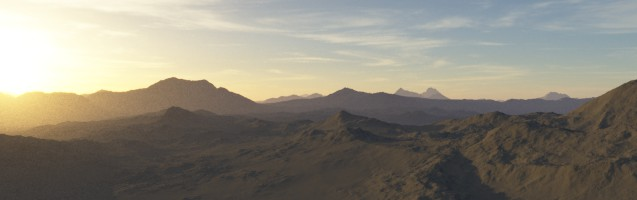## Introduction

Dynamic range has two parts to it. The range defines the smallest and largest values that can be quantified. When the quantifiable range is large, it is called high dynamic range (abbr. HDR). When it is low, it is called low dynamic range (abbr. LDR). Often these ranges are to large to experience both the low end and high end at the same time. We need time to adjust ourselves to accommodate the environment. For example, your vision goes black when you turn bright lights off in a room. After a moment, your eyes begin to adjust and you can make out details. This is the dynamic nature of our senses. Digital equipment works in the same way through exposure control, however it often records only a portion of the luminosity, making it impossible to recover details lost in the recording. When you work with HDR data, you have greater control over the luminosity and can apply a tone mapping algorithm to improve the dynamic range of the LDR image that gets rendered. This article explores the inner workings of HDR, what it's for, and how you can implement it to improve the quality of your renders.

## What is HDR?

In computer graphics, dynamic range refers to the luminosity of the scene. Luminosity values are encoded 0.0 for black, 1.0 for white, and anything in between as shades of grey. The problem is defining what is black and what is white. A light bulb is bright, but so is the sun. There's a significant difference in luminosity between the two that both cannot be visibly defined within a limited range. Take any camera for example and adjust the exposure settings.It's the same environment, but due to the limited dynamic range of the camera, you either show details in the sky at the expense of making everything else darker, or you brighten the subject at the expense of overexposing the sky. The problem comes after the fact. Once you take a photograph, what you see is what you get. This is where HDR comes into play. Our digital devices are limited in the ranges of luminosity they can display, so simply put our devices are in the low dynamic range. That doesn't mean your workflow should be limited to that range as well. HDR is not just about having a greater range of luminosity information to work with, it's also a process for post-processing the result according to your needs. This process is called tone mapping.The tone mapped image exposes more detail in the shadows and highlights, allowing you to see more of what was recorded.

## What is Tone Mapping?

HDR data by itself is not useful. We have a limited brightness range we can see with. What tone mapping does is compress the HDR image down into the low dynamic range in such a way to maximize detail. Any sort of compression can result in quality loss and in the case with tone mapping, it can create very surreal images. Sometimes this is done for artistic reasons, but generally a good tone mapping algorithm with careful use of its parameters will help you make the most of your HDR data.

### An Example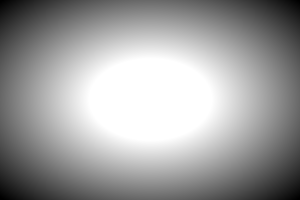Figure 1: LDR Image

The burned out (overexposed) centre in the LDR image is a result of only a portion of the range being visible. Let's take a look at the histogram.From left to right, the white line that divides the histogram into two parts is where the LDR ends. You can see that the rest of the image was clipped automatically to white. If you only had the LDR data, you would not be able to discover what lies beyond that point. With the HDR data, you can apply a tone mapping algorithm to compress the HDR data into an LDR image to expose as much detail as possible.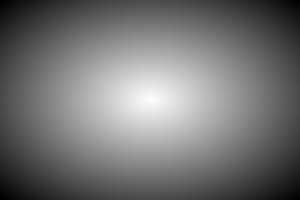Figure 2: HDR Tone Mapped Image

The beauty in HDR imaging lies in its configurability. Not only does it give you greater control over how the data gets compressed into LDR, but you can use this information to simulate the nature of our senses by auto adjusting the luminosity as you enter brighter or darker areas, known as auto exposure.

### Reinhard Tone Mapping

The following focuses on the minimal version of Reinhard's tone mapping algorithm. This version only factors in luminosity and does not discuss the post-recovery stage where dodge and burn is applied to the image to restore more detail. A link to his paper is provided in the references should you wish to learn more about this tone mapping algorithm.

Reinhard's tone mapping algorithm has 4 parts.

Equation 1

$L_w(x,y) = (0.2126 * red) + (0.7152 * blue) + (0.0722 * green)$

Where

$L_w(x,y)$ is the calculated luminance value for the pixel. This luminance formula is based on ITU-R BT.709, which empirically caters to the human eye. Since the human eye does not visualize red, blue, and green colours evenly, the typical greyscale calculation (R + G + B) / 3 would not be a good choice for calculating the pixel's luminosity.

Equation 2

$\bar{L}_w = exp(\frac{1}{N}\sum_{x,y} log(\delta + L_w(x,y)))$

This formula iterates over all the pixels in the image and calculates the average luminance, where

$\bar{L}_w$ is the calculated average luminance for the image. To perform this in hardware, you use mipmapping. By the time you reach a 1x1 pixel, you will have your average luminance value.

$\delta$ is a small adjustment made to handle pure blacks because log(0) = $-\infty$. If you are using the RGBE format to store your HDR data, note that the format does not support negative numbers and log(x), where 0 < x < 1 produces a negative value. The WebGL demo uses a delta of 1.0 and adjusts the exponent value later by -1.0 to restore the original value.

$L_w(x,y)$ is calculated in equation 1.

Equation 3

$L(x,y) = \frac{\alpha}{\bar{L}_w}L_w(x,y)$

Where

$L(x,y)$ is the calculated luminance scale for the pixel.

$\alpha$ is the key of the image, or rather a parameter that scales the exposure of the pixel. Small values (0.0 to 0.2) will underexpose the image and higher values (0.5 to 1.0) will tend to overexpose the image.

$\bar{L}_w$ is calculated in equation 2.

Equation 4

$L_d(x,y) = \frac{L(x,y)(1 + \frac{L(x,y)}{L^2_{white}})}{1 + L(x,y)}$

Where

$L_d(x,y)$ is the luminance scale you apply to your final pixel.

$L(x,y)$ is calculated in equation 3.

$L_{white}$ is the smallest luminance that will be mapped to pure white. By default, Reinhard sets this value to the maximum luminance in the scene, which avoids burn-out. It is still possible to simulate burn-out by modifying the key value in equation 3.

### Colour Space

The final step is to apply $L_d(x,y)$ to your pixel. As stated in equation 1, human vision does not map red, blue, and green colours evenly. So you cannot just apply this scale to the RGB colour directly. You must first convert the RGB pixel into CIE xyY space, where Y is the luminosity and xy is the chromaticity of the pixel. The conversion process is defined below.

Equation 5

Converting from RGB to XYZ to xyY.

$XYZ = \left[ \begin{array}{ccc} 0.4124 & 0.3576 & 0.1805 \\ 0.2126 & 0.7152 & 0.0722 \\ 0.0193 & 0.1192 & 0.9505 \end{array} \right] \left[ \begin{array}{ccc} R \\ G \\ B \end{array} \right]$ $xyY = \frac{X}{X + Y + Z}, \frac{Y}{X + Y + Z}, Y$

Equation 6

Once you have your xyY value, multiply your luminance scale as follows.

$xyY_Y = xyY_Y * L_d(x,y)$

Equation 7

Converting from xyY to XYZ to RGB.

$XYZ = (\frac{Y}{y}x, Y, \frac{Y}{y}(1.0 - x - y))$ $RGB = \left[ \begin{array}{ccc} 3.2406 & -1.5372 & -0.4986 \\ -0.9689 & 1.8758 & 0.0415 \\ 0.0557 & -0.2040 & 1.0570 \end{array} \right] \left[ \begin{array}{ccc} X \\ Y \\ Z \end{array} \right]$

## Implementation

### Step 1: Floating Point Textures

To implement HDR, you will need floating point texture support. You no longer need to clamp your pixels into the 0.0 and 1.0 range, just let them take any value. The sun in your game could have a pixel brightness of 1000 for instance.

Floating point textures do pose a problem. They consume 4 times the texture memory, some video cards are incapable of generating mipmaps with this format, and most importantly is that not all platforms support floating point textures. WebGL in particular only supports floating point textures as an extension, which means it's not guaranteed to be there if you use it. As an alternative, the RGBE format can be used. The WebGL demo that accompanies this article uses this format. Simply put, RGBE is a standard 32 bit image that stores compressed floating point RGB values. It was created by Gregory Larson for his Radiance renderer at a time when floating point textures were not feasible. RGBE does have its limitations though. RGBE compresses the floating point values, so there will be some quality loss. You also have to manually generate your mipmaps, and you have to manually filter the pixels. It can also create rings and bands as shown below.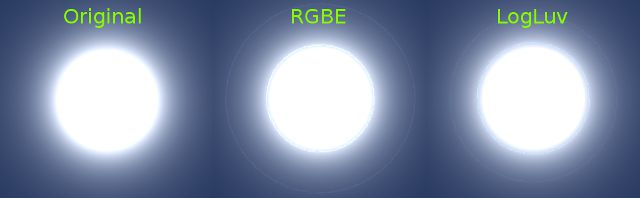Figure 3: HDR Encodings

You can see how RGBE and LogLuv (another format to store floating point pixels into a 32 bit image) can affect image quality. Most often though these defects will be covered up by the amount of other visual detail provided in your render.

Implementing HDR requires you to conform to a fully HDR pipeline. This means that if you use lightmaps, these textures must be in HDR format. You don't need to convert your standard colour textures, normal maps, or other such textures. If you don't use lightmaps, then you can ignore this step.

### Step 3: Create Two Floating Point Framebuffer Objects

The first framebuffer object will store the results of the rendered scene in an HDR texture. The second framebuffer object will store the log luminance values from your HDR texture. This data is required for the tone mapping stage. To calculate the log luminance, use a simple shader to calculate the log value of equation 1 for each pixel.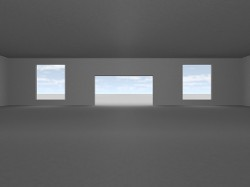->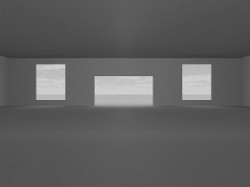Converting from HDR to log luminance.

### Step 4: Find the Average and Maximum Luminance

To find the average and maximum luminance values, you need to create a mipmap of your log luminance texture down to a 1x1 pixel that will contain your answer.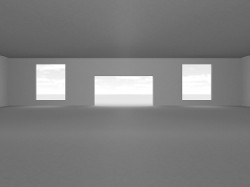->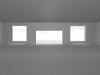->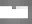->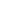Mipmapping the luminance texture

To find the average luminance, you bilinearly filter adjacent pixels until you reach a 1x1 pixel. To find the maximum luminance, you keep the pixel with the highest luminance value. This requires you to convert the RGB pixel into a floating point luminance value using equation 1. You then return the pixel with the highest luminance.

### Step 5: Tone Mapping

Apply Reinhard tone mapping to your HDR image. Substitute the average luminance calculated in step 4 into $\bar{L}_w$ and substitute the maximum luminance value into $L_{white}$. Adjust the key of the image as needed, or use a constant value throughout. It is also undesirable to set the average and maximum luminance values right away. It's better to create a delta and adjust the current values slowly until the new ones are met. This way when you turn around and go from a dark scene to a brighter one, the new exposure seeps in. This gives you that auto exposure look and feel.

## Conclusion

HDR improves the quality of your renders by better simulating human vision. With access to a higher range of luminosity data, you can apply a tone mapping algorithm to compress that data into an LDR image that provides the most detail. You also get a free auto-exposure system that better mimics how our vision reacts to varying light levels. HDR can be implemented using a platform independent format such as RGBE, but you are also free to take direct advantage of floating point textures that have been supported in mainstream video cards for quite some time. You just need to evaluate the expansive memory requirements in using them.

## References

1. Erik Reinhard. “Photographic Tone Reproduction for Digital Images ”. Retrieved 2013-02-05.

2. Wikipedia Editors (2013-01-29). “CIE 1931 color space”. Wikipedia. Retrieved 2013-02-05.

3. Wikipedia Editors (2013-01-07). “sRGB”. Wikipedia. Retrieved 2013-02-05.

The source code for this project is made freely available for download. The ZIP package below contains both the HTML and JavaScript files to replicate this WebGL demo.

The source code utilizes the Nutty Open WebGL Framework, which is an open sourced, simplified version of the closed source Nutty WebGL Framework. It is released under a modified MIT license, so you are free to use if for personal and commercial purposes.